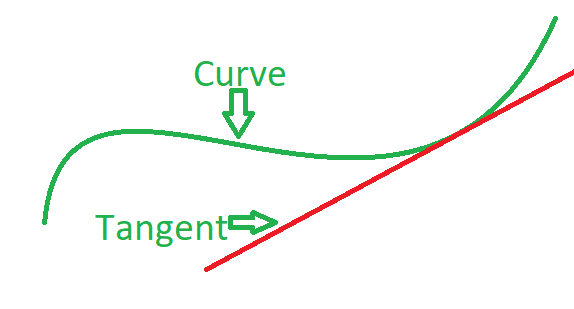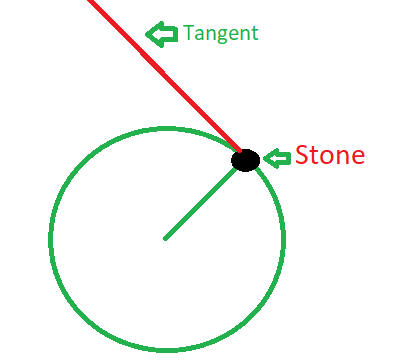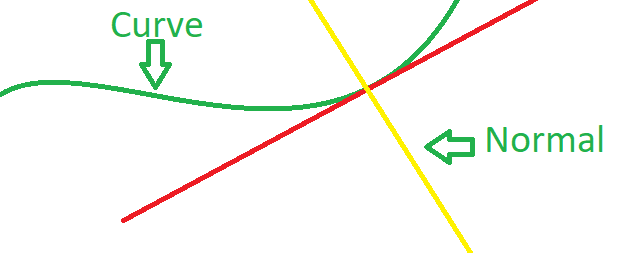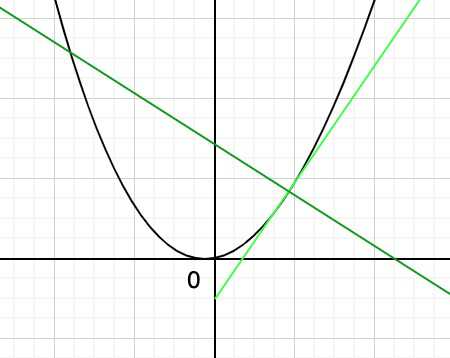Open in App
Not now

# Tangents and Normals

• Last Updated : 12 May, 2021

For a given curve tangent at a particular point is a straight line that just touches that curve at that point, and it goes in the same direction as the curve at that point, i.e if the curve stopped tracing the function at this point then it will go straight and that would be a tangent line.Let’s understand this by an example:

Imagine that you are rotating a stone in a circle that is tied to a thread.Now, if at any moment of time if you let go of the thread then the stone will follow a straight path, and that straight path is the tangent to the point of contact between the circular path and the stone when then stone is let go.

### Properties of a Tangent

1. Tangents just touch the curve at the point of contact.
2. If any tangent to a curve y = f(x) make angle θ with the x-axis, then dy/dx = slope of the tangent = tan θ.
3. If the slope of the tangent is zero, then tan θ will be equal to 0 and so θ = 0 which implies that the tangent line is parallel to the x-axis.
4. If θ = π/2, then tan θ will approach ∞, i.e., the tangent line is perpendicular to the x-axis.

### Applications of Tangents

1. If you are traveling by car around a corner and if you perform a drift then your car starts to skid, it will continue in a direction tangent to the curve.
2. If you hold a stone and swing it around in a circular motion and then let go, it will fly in a tangent to the circular motion.

Important Points:

• The length of the tangent is• The length of the subtangent is |y/dy/dx|
• When a tangent is parallel to line ax + by + c = 0, then dy/dx = -a/b.

Normals: A normal line to a curve is a straight line that is perpendicular to the tangent of that curve at a given point.For Example: Let’s take the curve y = x², if we want to draw the normal line at the point (1, 1), to the curve then we will draw the line tangent to the curve first at that point. Then we will draw a line perpendicular to the tangent. It will look like this.### Properties of a normal

1. A normal line at any point of a circle will always pass through the centre of the circle.
2. The normal to any curve is always perpendicular to the tangent at any point on the curve.

### Applications of normal

1. The centripetal force acting on a body that is moving in a circle is always normal to the point of contact at a given time
2. The spokes in a wheel are normal to the rim of a wheel at each point where the spoke connects with the centre.

### Equation of a Tangent and a Normal to a curve in the Cartesian Coordinates

At a point on the curve, the gradient of the curve is equal to the gradient of the tangent to the curve at that point. So, the equation of a tangent can be found by the gradient at that point to the curve and the given point as follows:

As we know that the equation of the straight line passes through point P (x0, y0) is

y – y0 = m(x – x0)

Here, m is the finite slope of the line. Now the slope of the tangent to a curve given is y = f(x) at point P (x0, y0) is f(x0)’. Then the equation of the tangent to the curve at point P(x0, y0) will be:

y – y0 = f(x0)'(x – x0)

For the normal, as we already know that the normal is always perpendicular to the tangent line. Then the slope of the normal to the curve will be:

-1/f(x0)’

So, the equation of the normal to the curve y = f(x) at the point (x0, y0) is :

y – y0 = [-1/f(x0)’](x – x0)

or

f(x0)'(y – y0) + (x – x0) = 0

### Equation of a Tangent and a Normal to a curve in Parametric Form

Let us assume the parametric form of the curve is

x = x(t)  ….(i)

y = y(t)  ….(ii)

Now we find the slope of the tangent to a curve at the point (x0, y0), by using the differentiation rule:

m = tan α = yt‘/xt

Hence, the equation of the tangent is:

y – y0 = yt‘/xt‘(x – x0)

Accordingly, the equation of the normal is:

y – y0 = – xt‘/yt‘(x – x0)

or

y’t(y – y0) + x’t(x – x0) = 0

### Equation of a Tangent and a Normal to a curve in Polar Coordinates

Let us assume the polar equation of the curve is r = f(θ). It represents the dependence of the radius vector r on the polar angle θ.  In the cartesian coordinates, this curve can be written in the following equations

x = r = f(θ) cosθ  ….(i)

y = r = f(θ)sinθ  ….(ii)

So, we get the parametric equation of the curve. Now we find the slope of the tangent to a curve at the point (x0, y0).

m = tanθ = y’θ/ x’θ  = r’θsin θ + rcosθ/r’θcosθ – rsinθ

Hence, the equation of the tangent is:

y – y0 = y’θ/ x’θ(x – x0)

Accordingly, the equation of the normal is:

y – y0 = – x’θ/y’θ(x – x0)

or

y’θ(y – y0) + x’θ(x – x0) = 0

### Sample Problems

Question 1. Find the slope of the tangent and the normal to the curve y = 6x2 – 10x at x = 1.

Solution:

The given curve is y = 6x2 – 10x

Now the gradient, dy/dx = 12x − 10

So, the slope of the tangent to the given curve at x = 1 is,

dy/dx]x=1 = 12 * 1 – 10 = 2

Slope of the normal will be:

= -1/2 = -0.5

Question 2. Find The slope of the tangent and normal to the curve y = 3x3 + 3sin(x) at x = 0.

Solution:

The given curve is y = 3x3 + 3sin(x)

Now the gradient, dy/dx = 9x2 + 3cos(x)

So, the slope of the tangent to the given curve at x = 0 is,

dy/dx]x=0 = 0 + 3 * 1 = 3

The slope of the normal will be:

= -1/3

Question 3. Find the equation of tangent to the curve y = 6x2 – 2x + 3 at P(1, 0).

Solution:

The given curve is y = 6x2 – 2x + 3

Now the gradient, dy/dx = 12x – 2

So, the slope of the tangent to the given curve at P(1,0) is

dy/dx]1,0 = 12 – 2 = 10

The equation of the line will be:

y – 0 = 10(x – 1)

y = 10x – 10

Question 4. Determine the point on the curve y = 6x2 – 8x + 1 where the tangent is parallel to the line y = 4x – 5.

Solution:

The given curve is y = 6x2 – 8x + 1

Now the gradient, dy/dx = 12x – 8

Tangent is parallel to y = 4x – 5

so,

12x0 – 8 = 4

or x0 = 1

Putting x = 1 in equation of the curve

We get,

y0 = 6 – 8 + 1

y0 = -1

So the point is (1, -1)

Question 5. Find the slope of the tangent to the curve given by:

x = sin2u, y = cos2u at point where u = π/2.

Solution:

Given:

x = psin3u …(i)

y = qcos3u …(ii)

The value of u = π/2

On differentiating eq(i) and (ii), w.r.t u, we get

dx/du = 3psin2u.cosu …(iii)

dy/du = -3qcos2u.sinu …(iv)

Now we find the slope of the tangent at point u = π/2dy/dx = -qcosu/psinu

dy/dx]u=π/2 = -qcos(π/2)/psin(π/2) = 0

Hence, the equation of tangent is y = 0.

My Personal Notes arrow_drop_up